# Electrostatics, Magnetism & Circuits Flashcards Preview

## AP Physics > Electrostatics, Magnetism & Circuits > Flashcards

Flashcards in Electrostatics, Magnetism & Circuits Deck (104):
1

2

3

4

5

6

7

8

9

10

11

12

13

14

15

16

17

## What concept and direction describes the path of motion that a positive test charge will travel, when put next to a stationary positive charge?

### Electrostatic field lines.  By convention, all positive charges have field lines with arrows pointing outwards, since a positive test charge will be repulsed.18

## What concept and direction describes the path of motion that a positive test charge will travel, when put next to a stationary negative charge?

### Electrostatic field lines.  By convention, all negative charges have field lines with arrows pointing inwards, since a positive test charge will be attracted.19

## Describe the motion of a positive test charge, if it's placed exactly at the midpoint between two equal positive stationary charges.

### It will remain immobile. The two positive charges repel the test positive charge equally, hence cancelling each other's force vector. There is no net force on the test charge.20

## What direction will a positive charge move, when introduced into a uniform electric field pointing directly upwards?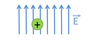### Directly upwards. A positive charge always follows the electric field.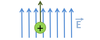21

## What direction will a negative charge move, when introduced into a uniform electric field pointing directly upwards?### Directly downwards. A negative charge always goes exactly opposite to the field lines.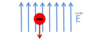22

## What direction will a non-charged particle move, when introduced into a uniform electric field pointing directly upwards?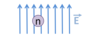### No motion. A non-charged particle (neutral) will not be accelerated in any direction by an electric field.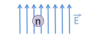23

## What direction will a proton move, when introduced into a uniform electric field pointing towards the right?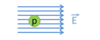### To the right. Positive charges always move in the direction of field lines.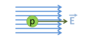24

## What direction will an electron move, when introduced into a uniform electric field pointing towards the left?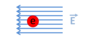### To the right. Negative charges move in the exact opposite direction of field lines.25

26

27

28

29

30

31

32

33

## What concept describes the set of positions of equal energy that a positive test charge can be placed, when put next to a stationary charge?

### Equipotential lines. All charges have equipotential lines that form closed, non-overlapping loops around them.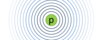34

35

## an electric dipole

### An electric dipole occurs as the result of any distinct separation of positive and negative charge in an object or system.  On the AP Physics exam, the simplest case is always assumed: a linear object with one positive and one negative end.36

## Describe the motion of a dipole placed into an electric field.### The dipole will rotate so that the positive end is aligned with the electric field and the negative end is exactly opposite to the field. Assuming that the dipole is overall neutral, there will not be any translation in the field.37

38

39

40

41

42

43

44

45

46

47

48

49

50

51

52

53

54

55

56

57

58

59

60

61

## What does this symbol stand for in a circuit diagram?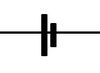### This is a battery. Batteries will always have a larger positive terminal (labeled +) and a smaller negative terminal (labeled –). Conventional current always flows from + to – around the circuit.62

## What does this symbol stand for in a circuit diagram?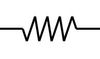### This is a resistor. Resistors impede current flow and have units of ohms.63

## What does this symbol stand for in a circuit diagram?### This is a capacitor. Capacitors store charge and have units of farads.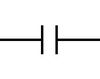64

## What does this symbol stand for in a circuit diagram?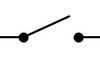### This is a switch. Switches can be open (as shown) so that no current flows around the circuit, or closed so that both dots are connected and current flows.65

## What does this symbol stand for in a circuit diagram?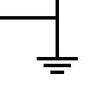### This is the symbol for ground. Ground is the physical end of the circuit path and represents zero current or zero voltage. If no ground symbol is given, the negative terminal of the battery is assumed to be ground.66

## What is the difference visually between resistors in parallel vs. series in a circuit?

### Resistors in parallel are each on a diverging circuit pathway. A single charge will pass through either one or the other to complete the circuit. (first image) Resistors in series follow each other on continuous circuit pathway. A single charge must go through both to complete the circuit. (second image)67

## What is the formula for calculating the total resistance of a circuit, for resistors in parallel?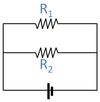### 1/RTotal = 1/R1 + 1/R2 + ... Where: RTotal = total resistance in Ω R1 = first resistor in Ω R2 = second resistor in Ω ... = any additional resistors in similar fashion68

## What is the total resistance in the circuit below?### RTotal = 5 Ω 1/RTotal = 1/R1 + 1/R2 = 1/10 + 1/10 = 2/10 = 1/569

## What is the formula for calculating the total resistance of a circuit, for resistors in series?### RTotal = R1 + R2 + ... Where: RTotal = total resistance in Ω R1 = first resistor in Ω R2 = second resistor in Ω ... = any additional resistors in similar fashion70

## What is the total resistance in the circuit below?### RTotal = 10 Ω RTotal = R1 + R2  = 4 + 6 = 1071

## What is the total resistance in the circuit below?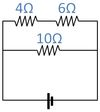### The total resistance is 5Ω. First find resistance for the 4Ω and 6Ω in series. Rseries = 4 + 6 = 10Ω. Then find resistance for the 10Ω and 10Ω in parallel. 1/RTotal = 1/10 + 1/10 = 2/10 = 1/5. RTotal = 5Ω.72

## What amount of current must be flowing around the circuit below?### The current is 2A. First find resistance for the 4Ω and 6Ω in series. Rseries = 4 + 6 = 10Ω. Then find resistance for the 10Ω and 10Ω in parallel. 1/RTotal = 1/10 + 1/10 = 2/10 = 1/5. RTotal = 5Ω. Finally, from Ohm's law: I = V/R = 10/5 = 2A73

## What amount of current must be flowing around the circuit below?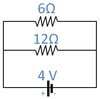### The current is 1A. First find resistance for the 12Ω and 6Ω in parallel. 1/RTotal = 1/12 + 1/6 = 3/12 = 1/4. RTotal = 4Ω. Then, from Ohm's law: I = V/R = 4/4 = 1A.74

75

76

77

## What is the formula for calculating the total capacitance of a circuit, for capacitors in parallel?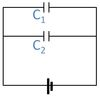### CTotal = C1 + C2 + ... Where: CTotal = total capacitance in F C1 = first capacitor in F C2 = second capacitor in F ... = any additional capacitors in similar fashion78

## What is the total capacitance in the circuit below?### Capacitance is 10F. CTotal = C1 + C2 = 8 + 2 = 10F79

## What is the formula for calculating the total capacitance of a circuit, for capacitors in series?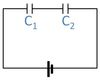### 1/CTotal = 1/C1 + 1/C2 + ... Where: CTotal = total capacitance in F C1 = first capacitor in F C2 = second capacitor in F ... = any additional capacitors in similar fashion80

## What is the total capacitance in the circuit below?### Capacitance is 2F. 1/CTotal = 1/C1 + 1/C2  = 1/4 + 1/4 = 2/4 = 1/2. CTotal = 2F.81

82

83

84

85

86

87

88

89

90

91

92

93

94

95

96

## RMS

### RMS stands for Root Mean Square, and represents the relationship between the instantaneous peak value and the average (mean) value. RMS can be calculated by taking the peak value and dividing by √2. In the image below, the peak current is 1A, so the RMS current will be 1/√2 = .7A97

98

99

100

101

102

## What is the amount of current flowing through the 10Ω resistor is the circuit as currently shown below?### Current is 1A through the 10Ω resistor. Since the switch is open in the circuit, no current flows through either the 4Ω or the 6Ω resistor. This means that the 10Ω resistor is the only one allowing current around the 10V circuit.  I = V/R = 10/10 = 1A103

## What voltage battery is necessary in order for 4A of current to be flowing through the circuit below?### Voltage must be 24V. First, find total resistance: 1/RTotal = 1/R1 + 1/R2 = 1/8 + 1/24 = 4/24 = 1/6. RTotal = 6Ω Then, From Ohm's law: V=IR = 4*6 = 24V.104

## In the circuit below, the total amount of current flowing is 1A. If both the 8Ω and 6Ω resistors were removed and the same battery used, by what factor will the current be changed?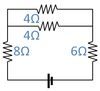### The new current will be 8x increased. Original resistance was R = 8 + 1/(1/4+1/4) + 6 = 8 + 2 + 6 = 16Ω.   I = V/R = V/16. New resistance is 1/(1/4+1/4) = 2Ω. I' = V/R' = V/2. Comparing I : I' = 1:8.